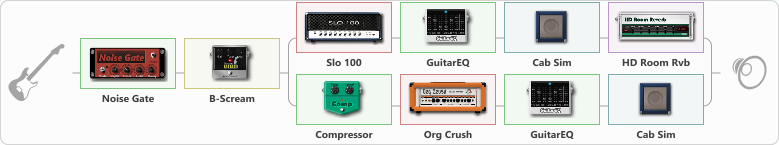# Soul of my Angel IsraeL

Discussion in 'ToneLib-GFX presets' started by Andy La Rosh, Mar 14, 2020.

1. Soul of my Angel IsraeL

Preset name: Soul of my Angel IsraeL

2 Hambs

Effects chain:Effect: "Noise Gate" (Dynamics / Filter), active - "yes"
{
"Mode" = Auto
"Depth" = 77
"Threshold" = 100
"Attack" = 138
"Hold" = 5
"Decay" = 5
}

Effect: "B-Scream" (Overdrive / Distortion), active - "yes"
{
"Drive" = 42
"Tone" = 77
"Level" = 41
}

Effect: "Splitter" (Dynamics / Filter), active - "yes"
{
"A-Bypass" = Off
"A-Pan" = 0
"A-Level" = 55
"B-Bypass" = Off
"B-Pan" = 0
"B-Level" = 55

'A' branch:
{

Effect: "Slo 100" (Amp simulators), active - "yes"
{
"Gain" = 60
"Bass" = 87
"Middle" = 22
"Treble" = 47
"Presence" = 40
"Master" = 52
"Level (dB)" = 6
}

Effect: "GuitarEQ" (Dynamics / Filter), active - "yes"
{
"160 Hz" = 6
"400 Hz" = -14
"800 Hz" = 2
"1.6 kHz" = 6
"3.2 kHz" = -4
"6.4 kHz" = -1
"12 kHz" = -15
"Level (dB)" = -3
}

Effect: "Cab Sim" (Cabinets), active - "yes"
{
"Model" = 4x12" Bluegrill
"Level (dB)" = 1
}

Effect: "HD Room Rvb" (Reverb), active - "yes"
{
"Time" = 3.6
"PreLPF" = 24
"PreDelay" = 71
"HiDamp" = 100
"LoGain" = -12.0
"Mix" = 41
}
}
'B' branch:
{

Effect: "Compressor" (Dynamics / Filter), active - "yes"
{
"Sense" = 38
"Level" = 50
}

Effect: "Org Crush" (Amp simulators), active - "yes"
{
"Gain" = 44
"Bass" = 61
"Middle" = 36
"Treble" = 43
"Presence" = 37
"Master" = 50
"Level (dB)" = 2
}

Effect: "GuitarEQ" (Dynamics / Filter), active - "yes"
{
"160 Hz" = 4
"400 Hz" = -13
"800 Hz" = 2
"1.6 kHz" = 6
"3.2 kHz" = -4
"6.4 kHz" = -1
"12 kHz" = -15
"Level (dB)" = -3
}

Effect: "Cab Sim" (Cabinets), active - "yes"
{
"Model" = 2x12" Silver Bell
"Level (dB)" = 1
}
}
}

Note: You will need to download and install the ToneLib-GFX software to use the preset.

File size:
1 KB
Views:
3,021